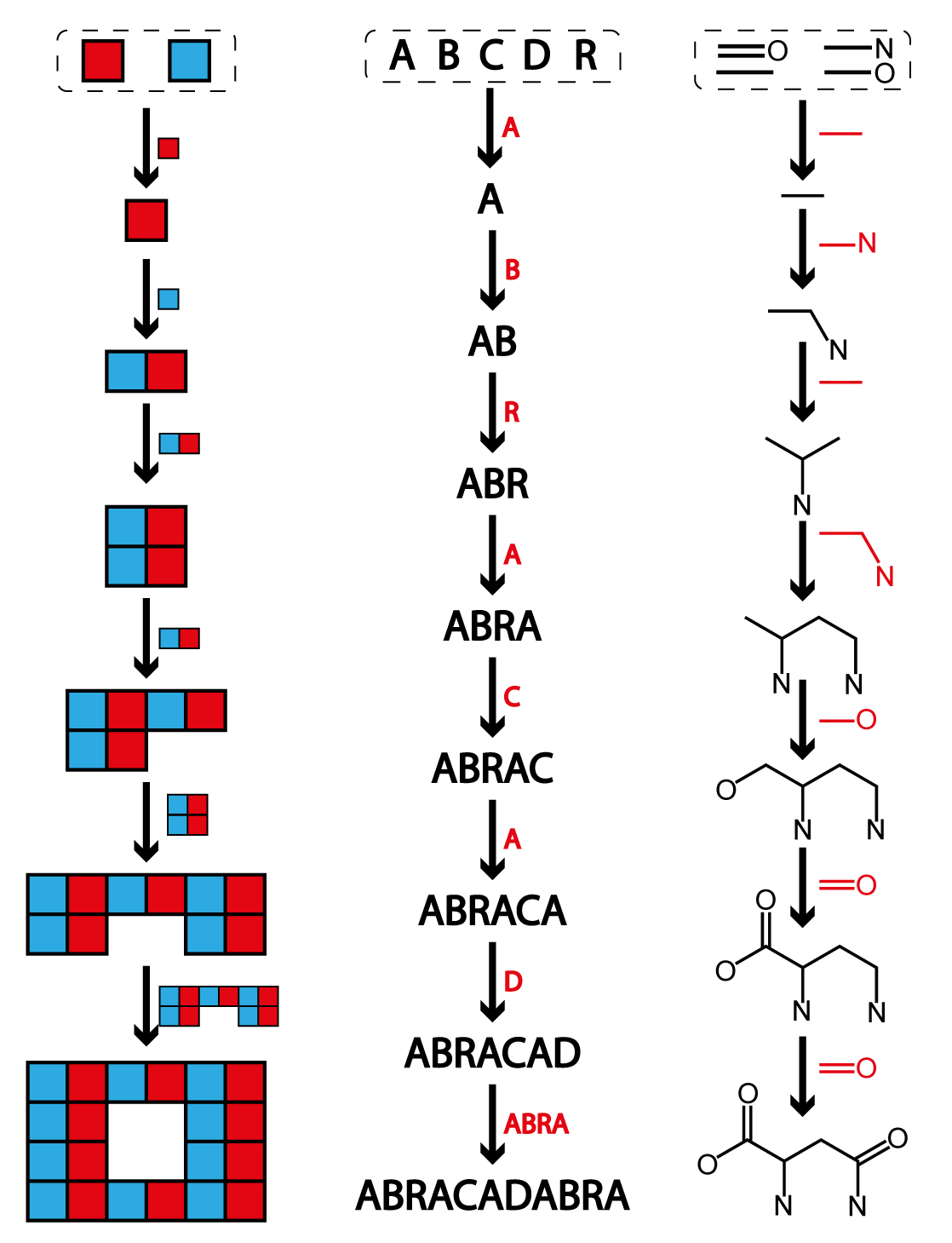# Understanding Assembly Indices

Calculating the Assembly Index of a molecule is an NP-Hard problem. We've developed a hierarchy of algorthmic approaches to this calculation. Use the descriptions below to understand the outputs from Molecular-Assembly.com.

## Molecular Assembly IndicesMolecular Assembly indices are a fundamental quantity in Assembly Theory because they measure the amount of information required to make a molecule. The Molecular Assembly Index (MA) of a molecule is defined as the fewest number of steps required to make the molecular graph by recursively using previously made structures. Let's unpack that statement a bit.

We when talk about the Molecular Assembly Index (MA) of a molecule we are talking about the steps required to make its graph structure, not the molecule itself. That means that we are not concerned with whether or not the operations we perform in this process could be physically done in a lab or a natural setting. Therefore some of the joining operations could seem counterintuitive to chemists or folks who make real molecules for a living. The number of steps required to make the graph will never be less than the number of individual mechanistic steps required to make the actual molecule. This is why we emphasize that we are calculating the fewest number of steps to make the molecular graph, not the molecule. When we compute the MA of a molecule we ignore the Hydrogen atoms. This is done primarily for computational simplicity because it significantly reduces the number of atoms in a molecule and has an insignificant effect on the structure of the compounds. Finally when we consider the basic building blocks of a molecule, we use the unique bonds in the molecule, not the atoms, as the components. That means that joining operations are defined by super imposing two (or more) atoms in different bonds.

Computing the MA of a compound is an NP-Hard problem, meaning that the comptuational time required to find the answer increases significantly with the size of the molecule. This is one reason we have decided to have Molecular-Assembly.com look-up precomputed MA values for molecules rather than compute them de-novo. In order to understand the data that this website provides it is important to understand the different algorithms we have developed to calculate MA. We detail the those algorithms below.

## Split Branch MA

In our 2021 paper entitled Identifying molecules as biosignatures with assembly theory and mass spectrometry we used an algorithm known as a the Split Branch algorithm, which provides an upper bound on the MA of a compound. This means that the MA of the compound is no higher than the value provided by this algorithm. This algorithm was chosen because the algorithm is both simpler and often faster than an exact calculation of the MA. The split branch algorithm computes the MA by first identiying large substructures with little overlap in the target graph, and then computes the MA of those structures independently. This means that if the target is partitioned into two different structures, some motifs that appear in both might be over-counted, resulting in an MA that is higher than the fewest number of steps required to construct the graph. This over-counting will not affect all molecules equally, it will depend on the structure of the molecule itself. We have provided an example of how this algorithm computes the MA of ATP in the figure below. A detailed description of this algorithm can be found in that paper and the associated supplement.